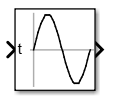# Sine Wave Function

• 库：

•## 描述

Sine Wave Function 模块输出正弦曲线波形。此模块可在基于时间或基于采样的模式下运行。

### 基于时间的模式

`$y=amplitude×\mathrm{sin}\left(frequency×time+phase\right)+bias.$`

• `0`（默认值）将使得模块在连续模式下运行。

• `>0` 将使得模块在离散模式下运行。

`$\begin{array}{l}\mathrm{sin}\left(t+\Delta t\right)=\mathrm{sin}\left(t\right)\mathrm{cos}\left(\Delta t\right)+\mathrm{sin}\left(\Delta t\right)\mathrm{cos}\left(t\right)\\ \mathrm{cos}\left(t+\Delta t\right)=\mathrm{cos}\left(t\right)\mathrm{cos}\left(\Delta t\right)-\mathrm{sin}\left(t\right)\mathrm{sin}\left(\Delta t\right)\end{array}$`

`$\left[\begin{array}{c}\mathrm{sin}\left(t+\Delta t\right)\\ \mathrm{cos}\left(t+\Delta t\right)\end{array}\right]=\left[\begin{array}{cc}\mathrm{cos}\left(\Delta t\right)& \mathrm{sin}\left(\Delta t\right)\\ -\mathrm{sin}\left(\Delta t\right)& \mathrm{cos}\left(\Delta t\right)\end{array}\right]\left[\begin{array}{c}\mathrm{sin}\left(t\right)\\ \mathrm{cos}\left(t\right)\end{array}\right]$`

`$\left[\begin{array}{cc}\mathrm{cos}\left(\Delta t\right)& \mathrm{sin}\left(\Delta t\right)\\ -\mathrm{sin}\left(\Delta t\right)& \mathrm{cos}\left(\Delta t\right)\end{array}\right]$`

1. 在 Sine Wave 模块对话框中，将时间设置为“`使用外部信号`”，这样模块图标上将出现一个输入端口。

2. 使用 Digital Clock 模块将一个时钟信号连接到此输入端口上。

3. 将时钟信号的采样时间设置为 Sine Wave 模块的采样时间。

`sin()` 数学模块库函数单独计算每个时间步的模块输出，从而可以避免舍入误差的累积。

### 基于采样的模式

`$y=A\mathrm{sin}\left(2\pi \left(k+o\right)/p\right)+b$`
• A 是正弦波的幅值。

• p 是每个正弦波周期内的时间采样数。

• k 为重复的整数值，范围从 0 到 p–1。

• o 是信号的偏移量（相位偏移）。

• b 是信号偏差。

## 参数

#### 编程用法

 模块参数：`SineType` 类型：字符向量 值：`'Time based'` | `'Sample based'` 默认值：`'Time based'`

#### 编程用法

 模块参数：`TimeSource` 类型：字符向量 值：`'Use simulation time'` | `'Use external signal'` 默认值：`'Use external signal'`

#### 编程用法

 模块参数：`Amplitude` 类型：字符向量 值：标量 默认值：`'1'`

#### 编程用法

 模块参数：`Bias` 类型：字符向量 值：标量 默认值：`'0'`

#### 编程用法

 模块参数：`Frequence` 类型：字符向量 值：标量 默认值：`'1'`

#### 编程用法

 模块参数：`Phase` 类型：字符向量 值：标量 默认值：`'0'`

#### 编程用法

 模块参数：`Samples` 类型：字符向量 值：标量 默认值：`'10'`

#### 编程用法

 模块参数：`Offset` 类型：字符向量 值：标量 默认值：`'0'`

#### 编程用法

 模块参数：`SampleTime` 类型：字符向量 值：标量 默认值：`'0'`

#### 编程用法

 模块参数：`VectorParams1D` 类型：字符向量 值：`'off'` | `'on'` 默认值：`'on'`

## 模块特性

 数据类型 `double` 直接馈通 `是` 多维信号 `否` 可变大小信号 `否` 过零检测 `否`